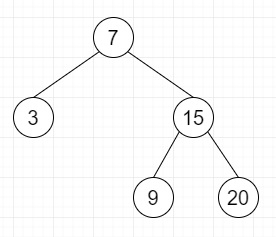# Construct a Binary Search Tree from given postorder in Python

Suppose we have the postorder traversal sequence of a binary search tree. We have to generate the tree from these sequences. So, if the postorder sequences is [9,15,7,20,3], then the tree will be −To form a tree we need inorder traversal also, but for binary search tree, the inorder traversal will be in the sorted form.

Let us see the steps −

• Inorder = sorted list of postorder traversal.

• Define a method build_tree(), this will take inorder, postorder −

• If inorder list is not empty −

• root := make a tree node with the last value of postorder, then delete that element

• ind := index of root data in inorder list

• right of root := build_tree(inorder from index ind to end, postorder)

• left of root := build_tree(inorder from 0 to index ind - 1, postorder)

• return root

## Example

Let us see the following implementation to get better understanding −

Live Demo

class TreeNode:
def __init__(self, data, left = None, right = None):
self.data = data
self.left = left
self.right = right
def print_tree(root):
if root is not None:
print_tree(root.left)
print(root.data, end = ', ')
print_tree(root.right)
class Solution(object):
def buildTree(self, inorder, postorder):
if inorder:
root = TreeNode(postorder.pop())
ind = inorder.index(root.data)
root.right = self.buildTree(inorder[ind+1:],postorder)
root.left = self.buildTree(inorder[:ind],postorder)
return root
ob1 = Solution()
postorder = [3,9,20,15,7]
inorder = list(sorted([3,9,20,15,7]))
print_tree(ob1.buildTree(inorder, postorder))

## Input

[9,3,15,20,7]
[9,15,7,20,3]

## Output

[3,7,9,15,20]